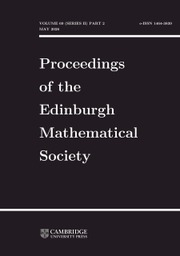Home
Hostname: page-component-5c569c448b-hlvcg Total loading time: 0.19 Render date: 2022-07-06T05:03:25.331Z Has data issue: true Feature Flags: { "shouldUseShareProductTool": true, "shouldUseHypothesis": true, "isUnsiloEnabled": true, "useRatesEcommerce": false, "useNewApi": true } hasContentIssue trueProceedings of the Edinburgh Mathematical Society

# AN INVERSE THEOREM FOR THE GOWERS $U^3(G)$ NORM

Published online by Cambridge University Press:  04 February 2008

## Abstract

HTML view is not available for this content. However, as you have access to this content, a full PDF is available via the ‘Save PDF’ action button.

There has been much recent progress in the study of arithmetic progressions in various sets, such as dense subsets of the integers or of the primes. One key tool in these developments has been the sequence of Gowers uniformity norms $U^d(G)$, $d=1,2,3,\dots$, on a finite additive group $G$; in particular, to detect arithmetic progressions of length $k$ in $G$ it is important to know under what circumstances the $U^{k-1}(G)$ norm can be large.

The $U^1(G)$ norm is trivial, and the $U^2(G)$ norm can be easily described in terms of the Fourier transform. In this paper we systematically study the $U^3(G)$ norm, defined for any function $f:G\to\mathbb{C}$ on a finite additive group $G$ by the formula

We give an inverse theorem for the $U^3(G)$ norm on an arbitrary group $G$. In the finite-field case $G=\mathbb{F}_5^n$ we show that a bounded function $f:G\to\mathbb{C}$ has large $U^3(G)$ norm if and only if it has a large inner product with a function $e(\phi)$, where $e(x):=\mathrm{e}^{2\pi\ri x}$ and $\phi:\mathbb{F}_5^n\to\mathbb{R}/\mathbb{Z}$ is a quadratic phase function. In a general $G$ the statement is more complicated: the phase $\phi$ is quadratic only locally on a Bohr neighbourhood in $G$.

As an application we extend Gowers's proof of Szemerédi's theorem for progressions of length four to arbitrary abelian $G$. More precisely, writing $r_4(G)$ for the size of the largest $A\subseteq G$ which does not contain a progression of length four, we prove that

$$r_4(G)\ll|G|(\log\log|G|)^{-c},$$

where $c$ is an absolute constant.

We also discuss links between our ideas and recent results of Host, Kra and Ziegler in ergodic theory.

In future papers we will apply variants of our inverse theorems to obtain an asymptotic for the number of quadruples $p_1\ltp_2\ltp_3\ltp_4\leq N$ of primes in arithmetic progression, and to obtain significantly stronger bounds for $r_4(G)$.

## Keywords

Type
Research Article
InformationYou have Access
68
Cited by

# Save article to Kindle

Note you can select to save to either the @free.kindle.com or @kindle.com variations. ‘@free.kindle.com’ emails are free but can only be saved to your device when it is connected to wi-fi. ‘@kindle.com’ emails can be delivered even when you are not connected to wi-fi, but note that service fees apply.

Find out more about the Kindle Personal Document Service.

AN INVERSE THEOREM FOR THE GOWERS $U^3(G)$ NORM
Available formats
×

# Save article to Dropbox

To save this article to your Dropbox account, please select one or more formats and confirm that you agree to abide by our usage policies. If this is the first time you used this feature, you will be asked to authorise Cambridge Core to connect with your Dropbox account. Find out more about saving content to Dropbox.

AN INVERSE THEOREM FOR THE GOWERS $U^3(G)$ NORM
Available formats
×

# Save article to Google Drive

To save this article to your Google Drive account, please select one or more formats and confirm that you agree to abide by our usage policies. If this is the first time you used this feature, you will be asked to authorise Cambridge Core to connect with your Google Drive account. Find out more about saving content to Google Drive.

AN INVERSE THEOREM FOR THE GOWERS $U^3(G)$ NORM
Available formats
×
×